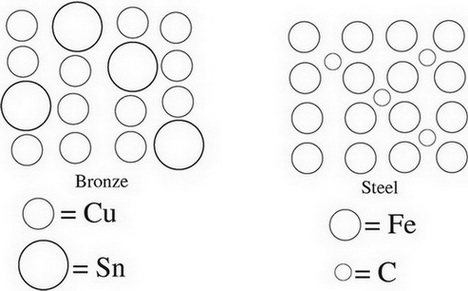# AP Chemistry Practice Test 5

### Test Information12 questions18 minutes

1. A proposed mechanism for a reaction is as follows:

NO2 + F2 →NO2F + F Slow step

F + NO2 → NO2F Fast step

What is the order of the overall reaction?

2. Starting with a stock solution of 18.0 M H2SO4, what is the proper procedure to create a 1.00 L sample of a 3.0 M solution of H2SO4 in a volumetric flask?

3. The reaction shown in the following diagram is accompanied by a large increase in temperature. If all molecules shown are in their gaseous state, which statement accurately describes the reaction?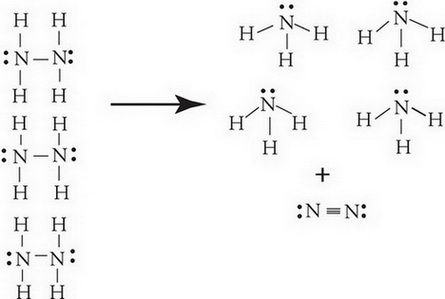4. A student mixes equimolar amounts of KOH and Cu(NO3)2 in a beaker. Which of the following particulate diagrams correctly shows all species present after the reaction occurs?

`Questions 5-7 refer to the following information.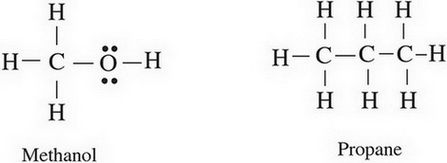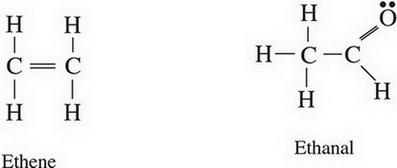`

5. Based on the strength of the intermolecular forces in each substance, estimate from greatest to smallest the vapor pressures of each substance in liquid state at the same temperature.

6. When in liquid state, which two substances are most likely to be miscible with water?

7. Between propane and ethene, which will likely have the higher boiling point and why?

8. 4NH3(g) + 5O2(g) → 4NO(g) + 6H2O(g)

The above reaction will experience a rate increase by the addition of a cataylst such as platinum. Which of the following best explains why?

9. The graph below shows the amount of potential energy between two hydrogen atoms as the distance between them changes. At which point in the graph would a molecule of H2 be the most stable?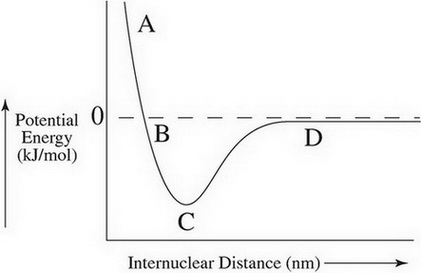10. N2(g) + O2(g) + Cl2(g) ↔ 2NOCl(g) Δ = 132.6 kJ/mol

For the equilibrium above, what would happen to the value of Δ if the concentration of N2 were to increase and why?

11. C(s) + 2S(s) → CS2(l) &DELTA;H = +92.0 kJ/mol

Which of the following energy level diagrams gives an accurate representation of the above reaction?

12. Two alloys are shown in the diagrams below—bronze, and steel. Which of the following correctly describes the malleability of both alloys compared to their primary metals?